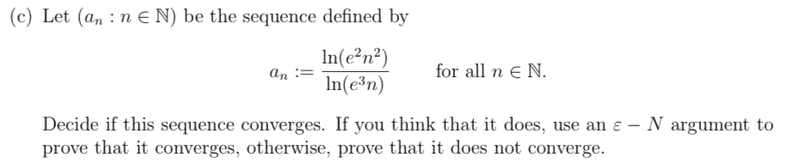# Does it converge?

UOAMCBURGER

## Homework Statement## Homework Equations

epsilon - N definition of convergence [/B]

## The Attempt at a Solution

Not sure how to determine whether this sequence converges or not. Thought it could have something to do with the fact that e^2 and e^3 are just constants, so when n > infinity those e terms become negligible in comparison. If you had to apply convergence definition how could you do that without L? [/B]

Staff Emeritus
Homework Helper
Gold Member

## Homework Statement

View attachment 232999

## Homework Equations

epsilon - N definition of convergence

## The Attempt at a Solution

Not sure how to determine whether this sequence converges or not. Thought it could have something to do with the fact that e^2 and e^3 are just constants, so when n > infinity those e terms become negligible in comparison. If you had to apply convergence definition how could you do that without L?
Do you know rules for logarithms ?

Such as ##\ \ln(a\cdot b) =\ln(a)+\ln(b)\,?##

What is ## \ \ln(e^2) \, ?##

UOAMCBURGER
Do you know rules for logarithms ?

Such as ##\ \ln(a\cdot b) =\ln(a)+\ln(b)\,?##

What is ## \ \ln(e^2) \, ?##
oh yes i do. Update: I used L'Hospital's Rule to find that the sequence converges to 2. But now separating the logarithms you get 2+ln(n^2)/3+ln(n), so would that change my answer I get using L'Hospital's rule? or can i assume an converges to 2 and then use the definition with L = 2?

Staff Emeritus
Homework Helper
Gold Member
oh yes i do. Update: I used L'Hospital's Rule to find that the sequence converges to 2. But now separating the logarithms you get 2+ln(n^2)/3+ln(n), so would that change my answer I get using L'Hospital's rule? or can i assume an converges to 2 and then use the definition with L = 2?
Did you mean ##\ \displaystyle \frac{2+\ln(n^2)}{3+\ln(n) } \,?##

If you write a "fraction" all on one line, you need to enclose the numerator and denominator each in parentheses; as in (2+ln(n^2))/(3+ln(n)) .

Also, you may further find that it helps to review rules for logarithms in general.

Another helpful one here is ##\ \displaystyle \ln(a^M) = M\ln(a)\ .##